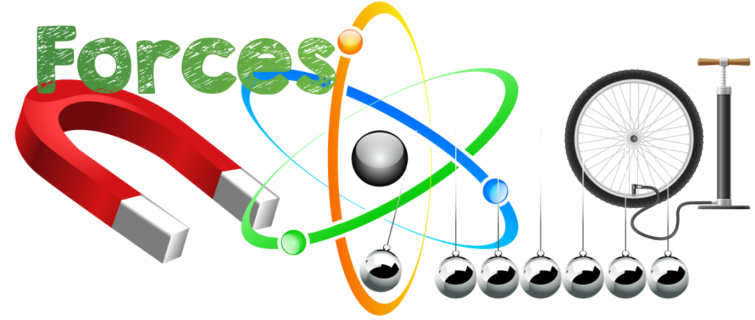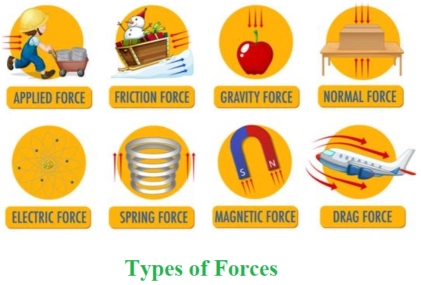Related Articles

# Contact and Non Contact Forces

• Difficulty Level : Easy
• Last Updated : 21 Jul, 2021

Any object which is pushed or pulled by force as a result of its contact with another object. Nothing can be pushed, halted, or changed direction without force. It is the quantitative interaction of two physical bodies, or an item and its surroundings. In nature, there are many sorts of forces.

You’re sitting in your room, reading this. Are you putting any force on the table right now? If that’s the case, what kind of force are you using? Taking a bottle, push it across the table. Have you just used force? What kind of pressure did you use? The bottle being pushed across the table is an example of ‘Applied Forces.’ Let’s take a closer look at the many sorts of forces.### Types of Forces

There are primarily two types of forces. They are:

• Contact forces
• Non-contact forces

### Contact Forces

The term “contact force” refers to any force that requires contact with another item. Contact forces are the basis of all mechanical forces.

Contact forces are further subdivided into the following categories:

(1) Muscular Force

Muscles work together to create a force that is known as muscular force. Muscle force only occurs when it comes into touch with anything. We use muscular force in our daily activities such as breathing, digesting, lifting a bucket, and dragging or pushing an object. Our task is made easier by using muscular force.

(2) Spring Force

Spring force is the force exerted by a compressed or stretched spring. Depending on how the spring is connected, the force generated might be a push or a pull.

(3) Frictional Force

The frictional force operates on an item when it changes its state motion. It’s the opposing force that arises when an object is moved or attempts to move a surface. Frictional force comes from contact between two surfaces and functions as a point of contact between two surfaces. The frictional force is used to ignite a matchstick or halt a moving ball, for example.

Friction has two types: sliding and static friction.

(4) Applied Force

When you push a table across the room, you apply a force that acts when it collides with something else. This is referred to as ‘applied force,’ which refers to a force that is applied to a person or object.

There are three types of applied force: push, pull and drag force.

(5) Normal Force

Even if a book appears to be stationary when it is placed on a table, it is not. The book is still being acted upon by an opposing force, that of gravity, which is pushing it towards the ground. This is known as the ‘normal force.’

(6) Tension Force

The force exerted by a completely stretched cable or wire attached to an object is known as tension. This creates a ‘tension force,’ which pulls in both directions and applies equal pressure.

(7) Air Resisting Force

When things move through the air, they are subjected to air resistive forces, which are frictional forces. These forces are inherently resistant.

### Non-Contact Forces

Non-contact forces are the sorts of forces that may be applied without making contact with any object.

They are further subdivided into the following forces:

(1) Gravitational Force

Newton’s law of gravity says that “gravitational forces between two bodies are directly equal to the product of their masses and inversely proportional to the square of the distance between them.” Large things, such as planets and stars, exert this force.

(2) Electrostatic Force

Electrostatic forces are the sorts of forces that all electrically charged bodies in the cosmos exert on other electrically charged substances. Based on the charge of the bodies, these forces can be both attractive and repulsive in nature.

(3) Magnetic Force

Magnetic forces are the sorts of forces exerted by a magnet on magnetic objects. They exist in the absence of any physical interaction between two things.### Balanced & Unbalanced Force

A balanced force is created when two forces are in opposite directions and have the same magnitude. Equilibrium is defined as equal and opposing forces operating on an item while it is not moving.

When two forces operating on a body are of uneven magnitude, this is known as an unbalanced force.

### Sample Problems

Problem 1: Which is the weakest force in nature?

Solution:

Gravity is the weakest force as its coupling constant is small in value.

Problem 2: Which force is strongest?

Solution:

The strongest force is the strong nuclear force which is 100 times stronger than the electromagnetic force.

Problem 3: What is the Line of Action of a Force?

Solution:

The line of action of the force is the path along which a force acts on an item. The point of application of the force is the location where the force acts on an item. The force of friction is the force that resists relative motion between the surfaces of two objects in contact and acts along the surfaces.

Problem 4: Give any evidence to show that there must be a force acting on earth and directed towards Sun?

Solution:

The sun rotates around the Earth. This fact requires us to consider a force that can generate the requisite centripetal acceleration to propel the rotation. The gravitational pull between the earth and the sun provides this essential force.

Problem 5: Is it mandatory for an object to move its place when we apply force on it?

Solution:

According to Newton’s first law of motion, the object tends to be in rest until any external force acts on it. However, the force must be unbalanced in nature, so it is not mandatory for an object to move when the force acts on it.

Attention reader! Don’t stop learning now. Join the First-Step-to-DSA Course for Class 9 to 12 students , specifically designed to introduce data structures and algorithms to the class 9 to 12 students

My Personal Notes arrow_drop_up